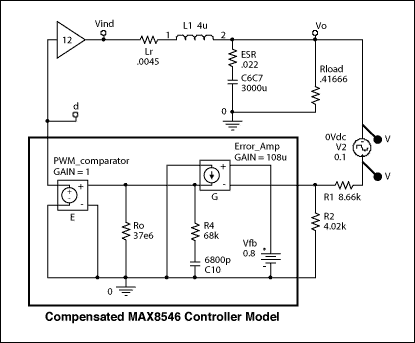# Simple PSPICE Model Predicts MAX8546 Stability and Transient Response

### Abstract

The MAX8546 is a low-cost, wide-input-voltage range, step-down controller with foldback current limit. A simple PSPICE model that can generate open-loop-gain Bode plots and predict load transient response for MAX8546 designs is presented.

The MAX8546 is a low-cost, wide-input-voltage range, step-down controller with foldback current limit. Its 300kHz switching frequency is well suited for use with low-cost external components such as powdered iron inductors and aluminum electrolytic capacitors, and still provides the necessary control bandwidth for good transient response. Low-cost, lossless overcurrent protection is achieved by utilizing the low-side MOSFET Rds(on). A detailed design procedure for the MAX8546 is presented in the MAX8546 data sheet.

Presented here is an additional design tool, a simple PSPICE model that can generate open-loop-gain Bode plots and predict load transient response for MAX8546 designs. The evaluation kit application circuit which is used for modeling is available for download. The model is simple enough to run on the evaluation version of PSPICE that can be downloaded.

Figure 1 shows the AC model for a step-down converter with a constant DC input voltage. The AC voltage driving the output L-C filter and load is the product of the DC input voltage, Vin, and the duty cycle perturbation, d, the output of the compensated controller.Figure 1. Model of step-down converter for AC analysis.

Figure 2 shows the PSPICE implementation of the AC model. The output voltage is divided down to a feedback threshold voltage, Vfb, of 0.8V by resistors R1 and R2. The transconductance error amplifier of the MAX8546 is modeled by a voltage-controlled current source, G, with a gain of 108µs, as specified in the data sheet. R4 and C10 form the compensation network connected to the MAC8546's COMP pin. The output resistance, Ro, of the transconductance amplifier is set at 37MΩ. The MAX8546 uses a 1V ramp for the PWM comparator, resulting in a PWM modulator small-signal gain of 1. The controller's output is multiplied by a gain equal to the input DC voltage (12V), and the resulting AC voltage drives the output L-C filter and load. Parasitic elements, such as output capacitor ESR and inductor series resistance, should be included as shown. The 100mV AC voltage source, V2, perturbs the loop for frequency domain AC analysis in PSPICE. The gain from the positive terminal of V2 to its negative terminal represents the open-loop gain. The Bode plot thus obtained is shown Figure 3. A phase margin of approximately 85 degrees is observed, indicating a well-damped system.Figure 2. PSPICE schematic for MAX8546 AC analysis.Figure 3. Simulated open-loop gain and phase for step-down converter.

The load transient response is obtained from time domain simulation of the PSPICE schematic shown in Figure 4. The AC source, V2, is removed for time domain analysis. The pulse current source, I2, simulates a load step of 3A. The slew rate for the load step is set at 15A/µs by setting the TR (rise time) and TF (fall time) parameters for I2. The initial conditions are set to their nominal values as shown, and PSPICE is instructed to skip bias point calculations by checking the appropriate box in the simulation settings dialog box. For the compensation capacitor, the initial condition is a voltage equal to the nominal duty cycle obtained as Vo/Vin. The simulated load transient response is shown in Figure 5. The well-damped output-voltage transient waveforms confirm the frequency response obtained from the AC model.Figure 4. PSPICE schematic for MAX8546 time domain analysis.Figure 5. Simulated load transient response.

The peak deviation, Vout , is 62mV and the settling time, Ts, is 30µs. Figure 6 shows actual load transient response measured on the MAX8546 evaluation kit. The results closely match those predicted by the model. The peak deviation measures approximately 65mV and the settling time is around 28µs.Figure 6. Measured load transient response.